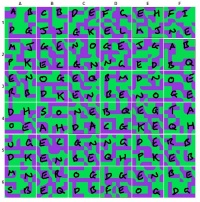# Be Amazed

(Difference between revisions)
;
 Revision as of 23:46, 25 June 2008 (edit)Sugarfiend06 (Talk | contribs)m (Well, don't need that anymore...)← Previous diff Revision as of 05:05, 26 June 2008 (edit) (undo)Sugarfiend06 (Talk | contribs) (Just adding CJWilkie's directions!)Next diff → Line 7: Line 7: A few phones received additional images, with the note: A few phones received additional images, with the note:
A couple extra for your trouble, clown. I remember favors...
A couple extra for your trouble, clown. I remember favors...
- * Attached are images, appearing like pieces to a puzzle (or a 'maze'?), labelled 1-6 and A-F (eg, 1A.jpg -> 6F.jpg) + + * Attached are images, appearing like pieces to a puzzle and/or maze, labelled 1-6 and A-F (eg, 1A.jpg -> 6F.jpg) ==== Pieces ==== ==== Pieces ==== +
{| cellpadding="0" cellspacing="2" width="1" {| cellpadding="0" cellspacing="2" width="1" |- |- Line 68: Line 70: |[[Image:amazed_6F.jpg|100px]] |[[Image:amazed_6F.jpg|100px]] |} |} - +
==The solution== ==The solution== There are 21 unique shapes in the puzzle. If you use the first shape as "A", the second as "B", and so forth, going from left to right, top to bottom, the following pattern appears: There are 21 unique shapes in the puzzle. If you use the first shape as "A", the second as "B", and so forth, going from left to right, top to bottom, the following pattern appears: - A B C B D E F G E H F I + {| class="quote" - D G J J G K E L M J N E + | - M J G E N O G E J G A B + A B C B D E F G E H F I
- P K E C G N N G D E B Q + D G J J G K E L M J N E
- E N O G E Q B M D N O E + M J G E N O G E J G A B
- R B D K E N B E N O G E + P K E C G N N G D E B Q
- D H S O N E B Q E G T A + E N O G E Q B M D N O E
- O E A H D A C G K E Q H + R B D K E N B E N O G E
- U G E C G N N G D E R B + D H S O N E B Q E G T A
- D K E N B E Q H P K E B + O E A H D A C G K E Q H
- M N E R O G D G E N B E + U G E C G N N G D E R B
- S B E Q D B F E O G D G + D K E N B E Q H P K E B
+ M N E R O G D G E N B E
+ S B E Q D B F E O G D G
+ |[[Image:Genius.jpg|200px]] + |} Then use the following substitution cypher: Then use the following substitution cypher:

## Be Amazed

• Joker cells began receiving texts on June 25th saying one of:
To find the next sideshow, share these with your fellow goons. Then be amazed.
To find the next sideshow, share these with your fellow goons. There's no substitution for fun.

A couple extra for your trouble, clown. I remember favors...
• Attached are images, appearing like pieces to a puzzle and/or maze, labelled 1-6 and A-F (eg, 1A.jpg -> 6F.jpg)

## The solution

There are 21 unique shapes in the puzzle. If you use the first shape as "A", the second as "B", and so forth, going from left to right, top to bottom, the following pattern appears:

 A B C B D E F G E H F I D G J J G K E L M J N E M J G E N O G E J G A B P K E C G N N G D E B Q E N O G E Q B M D N O E R B D K E N B E N O G E D H S O N E B Q E G T A O E A H D A C G K E Q H U G E C G N N G D E R B D K E N B E Q H P K E B M N E R O G D G E N B E S B E Q D B F E O G D GThen use the following substitution cypher:

From:

 A B C D E F G H I J K L M N O P Q R S T U

To:

 C O L R - M E I P S D J U T H N F W G A V

...to reveal this message:

```  color-me-imp
ressed-just-
use-the-seco
nd-letter-of
-the-fourth-
word-to-the-
right-of-eac
h-circled-fi
ve-letter-wo
rd-to-find-o
ut-where-to-
go-from-here
```
 Color me impressed. Just use the second letter of the fourth word to the right of each circled five-letter word to find out where to go from here

Using the third issue of The Hahaha Times, the next step was found at Whysoserious.com/Redballoons.

##### TDKR trails

May 2011
The Fire Rises
December 2011
Operation Early Bird

##### TDK trails

May 2007
IBIHD Too
July 2007
ComicCon
October 2007
Halloween
November 2007
Personality Profile
The Perfect Getaway
December 2007
Step Right Up
Taste/Theatrical
March 2008
IBIHD Campaign
April 2008
Clown Travel Agency
It's all part of the plan
Operation Slipknot
June 2008
Free Pizza
Laugh Til It Hurts
Be Amazed
July 2008
Operator